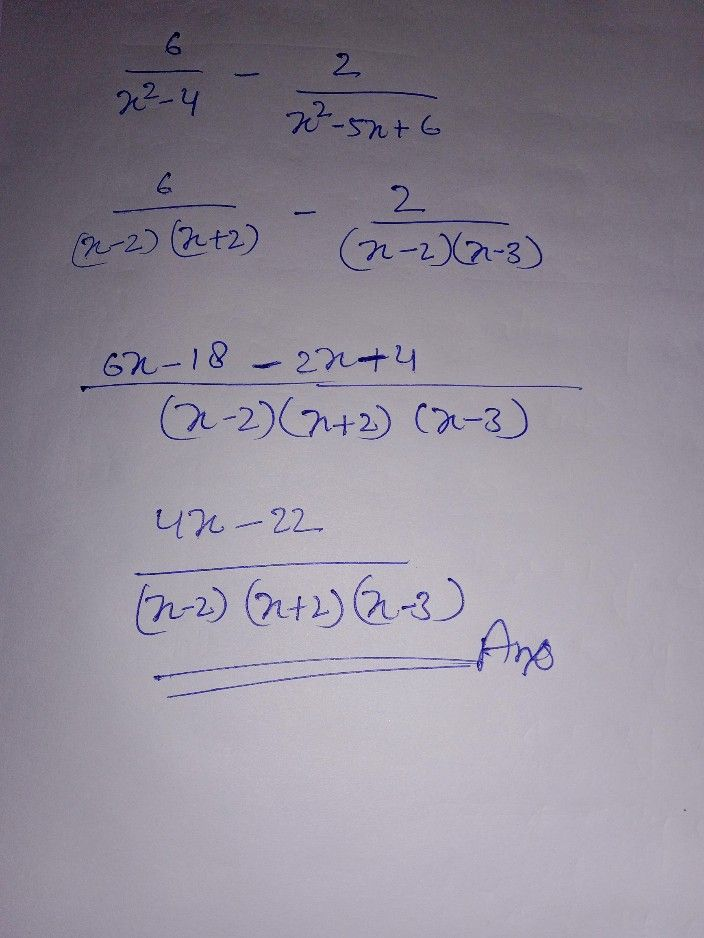Symbol
Problem4. To add and subtract rational expressions with unlike denominators you the following steps. Steps in adding or subtracting rational $\dfrac {6} {x^{2}-4}-\dfrac {2} {x^{2}-5x+6}$ expressions with previous unlike denominators Write $you7$ $hcrc$ $fon$ 1. Factor the $so11$ $+100$ denominator of $cacH$ $\dfrac {6} {\left(x-2\right)\left(x+2\right)}-$ $\dfrac {2} {\left(x-2\right)\left(x-3\right)}$ comparison. fraction to help find the $cD$ 2. Find the least common $LCD:\left(x-2\right)\left(x+2\right)\left(x-3\right)$ denominator $\left(LCD\right)$ $3$ Multiply each expression by its $\dfrac {6\left(LCD\right)} {\left(x-2\right)\left(x+2\right)}$ $\dfrac {2\left(LCD\right)} {\left(x-2\right)\left(x-3\right)}$ LCD $4$ Write the simplified $6\left(x-3\right)-2\left(x+2\right)$ expression. 5. Let the simplified expression as the numerator and the $\dfrac {6x-18-2x-4} {\left(x-2\right)\left(x+2\right)\left(x-3\right)}$ LCD as the denominator of the new fraction 6. Combine like terms and reduce the rational expression if you can. In this $\dfrac {4x-22} {\left(x-2\right)\left(x+2\right)\left(x-3\right)}$ case, the rational expression cannot be simplified.
10th-13th grade
Geometry
Search count: 116
SolutionQanda teacher - henrysee